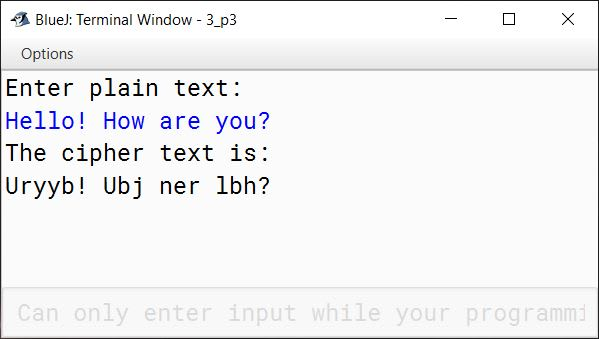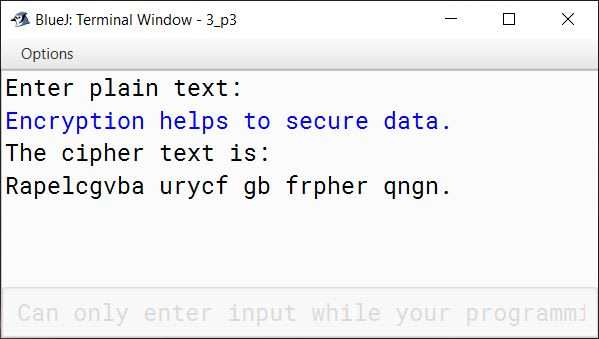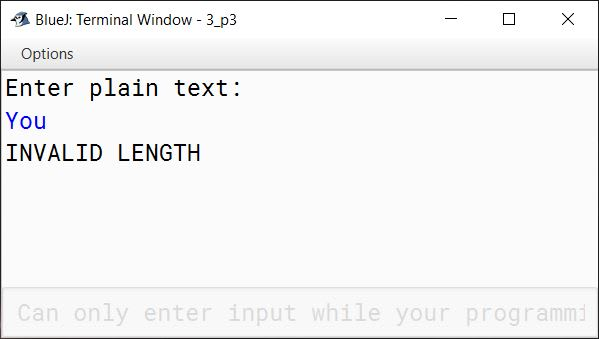# Solved 2017 Practical Paper ISC Computer Science

## Practical Questions

#### Question 1

A company manufactures packing cartons in four sizes, i.e. cartons to accommodate 6 boxes, 12 boxes, 24 boxes and 48 boxes. Design a program to accept the number of boxes to be packed (N) by the user (maximum up to 1000 boxes) and display the break-up of the cartons used in descending order of capacity (i.e. preference should be given to the highest capacity available, and if boxes left are less than 6, an extra carton of capacity 6 should be used.)

Test your program with the following data and some random data:

Example 1

INPUT:
N = 726

OUTPUT:
48 * 15 = 720
6 * 1 = 6
Remaining boxes = 0
Total number of boxes = 726
Total number of cartons = 16

Example 2

INPUT:
N = 140

OUTPUT:
48 * 2 = 96
24 * 1 = 24
12 * 1 = 12
6 * 1 = 6
Remaining boxes = 2 * 1 = 2
Total number of boxes = 140
Total number of cartons = 6

Example 3

INPUT:
N = 4296

OUTPUT:
INVALID INPUT

##### Solution
```import java.util.Scanner;

public class CartonBoxes
{
public static void main(String args[]) {
Scanner in = new Scanner(System.in);

System.out.print("Enter number of boxes (N): ");
int n = in.nextInt();

if (n < 1 || n > 1000) {
System.out.println("INVALID INPUT");
return;
}

int cartonSizes[] = {48, 24, 12, 6};

int total = 0;
int t = n;
for (int i = 0; i < cartonSizes.length; i++) {
int cartonCount = t / cartonSizes[i];
t = t % cartonSizes[i];
total += cartonCount;
if (cartonCount != 0) {
System.out.println(cartonSizes[i] + " * " + cartonCount +
" = " + (cartonSizes[i] * cartonCount));
}
}

/*
* This if check is for the case when
* boxes left are less than 6. We need
* one more carton of capacity 6 in this
* case so total is incremented by 1.
*/
if (t != 0) {
System.out.println("Remaining boxes = " + t
+ " * 1 = " + t);
total++;
}
else {
System.out.println("Remaining boxes = 0");
}

System.out.println("Total number of boxes = " + n);
System.out.println("Total number of cartons = " + total);
}
}```
##### Output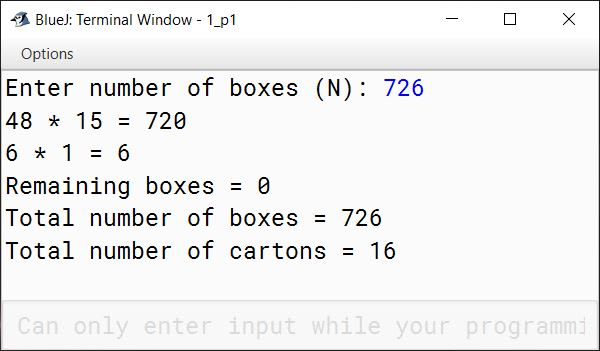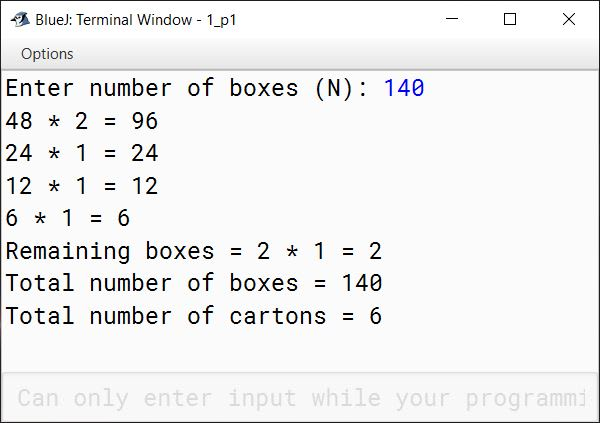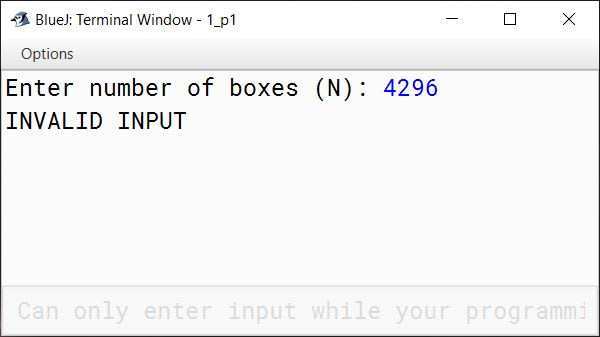#### Question 2

The result of a quiz competition is to be prepared as follows:

The quiz has five questions with four multiple choices (A, B, C, D), with each question carrying 1 mark for the correct answer. Design a program to accept the number of participants N such that N must be greater than 3 and less than 11. Create a double-dimensional array of size (Nx5) to store the answers of each participant row-wise. Calculate the marks for each participant by matching the correct answer stored in a single-dimensional array of size 5. Display the scores for each participant and also the participant(s) having the highest score.

Example: If the value of N = 4, then the array would be:

Q1Q2Q3Q4Q5
Participant 1ABBCA
Participant 3AABAC
Participant 4DCCAB
 Key to the question: D C C B A

Note: Array entries are line fed (i.e. one entry per line)

Test your program for the following data and some random data.

Example 1

INPUT:
N = 5
Participant 1 D A B C C
Participant 2 A A D C B
Participant 3 B A C D B
Participant 4 D A D C B
Participant 5 B C A D D
Key: B C D A A

OUTPUT:
Scores:
Participant 1 = 0
Participant 2 = 1
Participant 3 = 1
Participant 4 = 1
Participant 5 = 2
Highest Score:
Participant 5

Example 2

INPUT:
N = 4
Participant 1 A C C B D
Participant 2 B C A A C
Participant 3 B C B A A
Participant 4 C C D D B
Key: A C D B B

OUTPUT:
Scores:
Participant 1 = 3
Participant 2 = 1
Participant 3 = 1
Participant 4 = 3
Highest Score:
Participant 1
Participant 4

Example 3

INPUT:
N = 12

OUTPUT:
INPUT SIZE OUT OF RANGE.

##### Solution
```import java.util.Scanner;

public class QuizCompetition
{
public static void main(String args[]) {
Scanner in = new Scanner(System.in);
System.out.print("Enter the Number of Participants (N): ");
int n = in.nextInt();

if (n <= 3 || n >= 11) {
System.out.println("INPUT SIZE OUT OF RANGE.");
return;
}

char key[] = new char;

for (int i = 0; i < n; i++) {
System.out.println("Participant " + (i+1));
for (int j = 0; j < 5; j++) {
}
}

for (int i = 0; i < 5; i++) {
key[i] = in.next().charAt(0);
}

int hScore = 0;
int score[] = new int[n];

System.out.println("Scores:");
for (int i = 0; i < n; i++) {
for (int j = 0; j < 5; j++) {
score[i]++;
}
}

if (score[i] > hScore) {
hScore = score[i];
}

System.out.println("Participant " + (i+1) + " = " + score[i]);
}

System.out.println("Highest Score:");
for (int i = 0; i < n; i++) {
if (score[i] == hScore) {
System.out.println("Participant " + (i+1));
}
}
}
}```
##### Output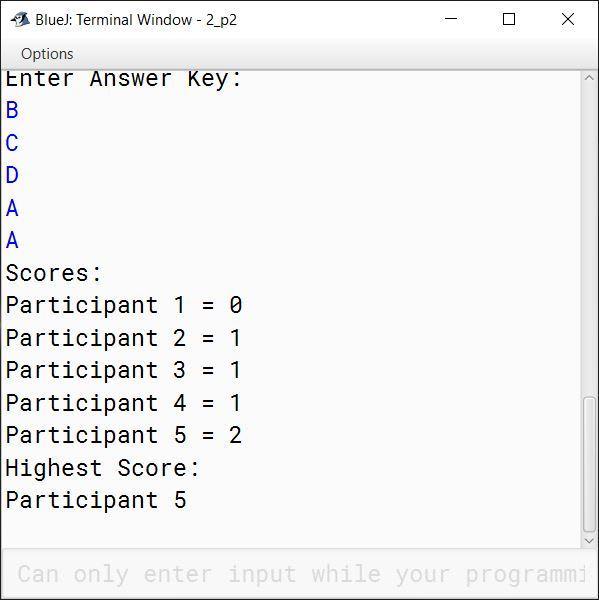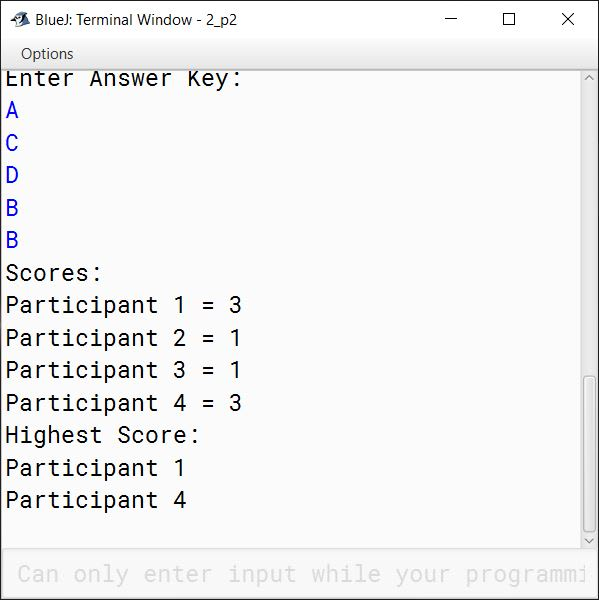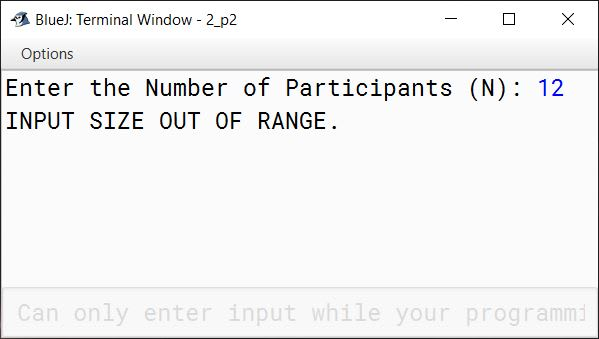#### Question 3

Caesar Cipher is an encryption technique which is implemented as ROT13 ('rotate by 13 places'). It is a simple letter substitution cipher that replaces a letter with the letter 13 places after it in the alphabets, with the other characters remaining unchanged.

ROT13

 A/a B/b C/c D/d E/e F/f G/g H/h I/i J/j K/k L/l M/m ↕ ↕ ↕ ↕ ↕ ↕ ↕ ↕ ↕ ↕ ↕ ↕ ↕ N/n O/o P/p Q/q R/r S/s T/t U/u V/v W/w X/x Y/y Z/z

Write a program to accept a plain text of length L, where L must be greater than 3 and less than 100.

Encrypt the text if valid as per the Caesar Cipher.

Test your program with the sample data and some random data.

Example 1

INPUT:
Hello! How are you?

OUTPUT:
The cipher text is:
Uryyb! Ubj ner lbh?

Example 2

INPUT:
Encryption helps to secure data.

OUTPUT:
The cipher text is:
Rapelcgvba urycf gb frpher qngn.

Example 3

INPUT:
You

OUTPUT:
INVALID LENGTH

##### Solution
```import java.util.Scanner;

public class CaesarCipher
{
public static void main(String args[]) {
Scanner in = new Scanner(System.in);
System.out.println("Enter plain text:");
String str = in.nextLine();
int len = str.length();

if (len <= 3 || len >= 100) {
System.out.println("INVALID LENGTH");
return;
}

StringBuffer sb = new StringBuffer();
for (int i = 0; i < len; i++) {
char ch = str.charAt(i);
if ((ch >= 'A' && ch <= 'M') || (ch >= 'a' && ch <= 'm')) {
sb.append((char)(ch + 13));
}
else if ((ch >= 'N' && ch <= 'Z') || (ch >= 'n' && ch <= 'z')) {
sb.append((char)(ch - 13));
}
else {
sb.append(ch);
}
}

String cipher = sb.toString();
System.out.println("The cipher text is:");
System.out.println(cipher);
}
}```
##### Output